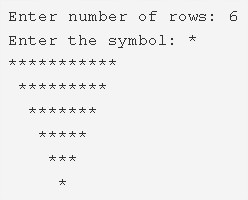# C Program Inverted Pyramid Star Pattern | 4 Ways – C Programs

C Program to print an inverted pyramid star pattern – In this article, we will detail in on the multiple ways to print an inverted pyramid star pattern in C programming.

Suitable examples and sample programs have also been added so that you can understand the whole thing very clearly. The compiler has also been added with which you can execute it yourself.

The ways mentioned in this distinct piece are as follows:

• Using For Loop
• Using While Loop
• Using Do-While Loop

As it is known to all, a pyramid is a three-dimensional figure which has a square as its base and it narrows off into a point giving it the height or the third dimension.

This is what an inverted pyramid looks like:As you can see, you will have to specify the number of rows. Subsequently, the pyramid will be printed accordingly taking the number of rows into consideration.

Thus, the different methods used to print an inverted pyramid star pattern in C programming are as follows:

## Using For Loop

1. Read the entered number, the entered a character using scanf, gectchar functions and store the values into the integer variable n, character variable ch.

2) The outer for loop iterates through rows with the structure for(i=n;i>0;i–).

3) The 1st inner for loop iterates through columns with the structure for(j=0;j<n-i;j++).

It prints space.

4) The 2nd inner for loop iterates through columns with the structure for(j=0;j<(i*2)-1;j++)

It prints the symbol which is entered by the user.

5) Cursor comes to the next line for each iteration of i value.

Output:

### Using While Loop

1. i=n, The outer while loop iterates through rows until the condition i>0 is false.

2)j=0, The 1st inner while loop iterates through columns until the condition j<n-i becomes false.

It prints space.

j value increased by 1.Again checks the condition.

3) j=0, The 2nd inner while loop iterates through columns until the condition j<(i*2)-1 becomes false.

It prints the symbol which is entered by the user.

j value increased by 1.Again checks the condition.

4) Cursor comes to next line.

5) i value decreased by 1. The outer while loop again checks the condition.

Output:

#### Using Do-While Loop

1. i=n, the outer do-while loop iterates through rows.

2) j=0, the 1st inner do-while loop iterates through columns.

It prints space.j value increased by 1.

Checks the condition j<n-i. If the condition is true then the 1st inner loop iterates again. Repeats until the condition becomes false.

3) j=0, the 2nd inner do-while loop iterates through columns.

It prints symbol,j value increased by 1.

Checks the condition j<i*2-1. If the condition is true then the 2nd inner loop iterates again. Repeats until the condition becomes false.

4) Cursor comes to next line.

5) i value decreased by 1.checks the condition i>0. If the condition is true the outer loop iterates again. Repeats until the condition becomes false.

Output:
x

## C Program To Print Number Of Days In A Month | Java Tutoring

C program to input the month number and print the number of days in that ...